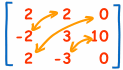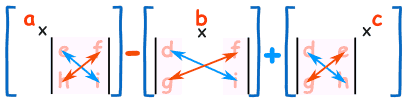# Inverse of a Matrix using Minors, Cofactors and Adjugate

(Note: also check out Matrix Inverse by Row Operations and the Matrix Calculator.)

We can calculate the Inverse of a Matrix by:

• Step 1: calculating the Matrix of Minors,
• Step 2: then turn that into the Matrix of Cofactors,
• Step 3: then the Adjugate, and
• Step 4: multiply that by 1/Determinant.

But it is best explained by working through an example!

## Example: find the Inverse of A:It needs 4 steps. It is all simple arithmetic but there is a lot of it, so try not to make a mistake!

## Step 1: Matrix of Minors

The first step is to create a "Matrix of Minors". This step has the most calculations.

For each element of the matrix:

Put those determinants into a matrix (the "Matrix of Minors")

### Determinant

For a 2×2 matrix (2 rows and 2 columns) the determinant is easy: ad-bc

 Think of a cross: Blue means positive (+ad), Red means negative (-bc)(It gets harder for a 3×3 matrix, etc)

### The Calculations

Here are the first two, and last two, calculations of the "Matrix of Minors" (notice how I ignore the values in the current row and columns, and calculate the determinant using the remaining values):And here is the calculation for the whole matrix:## Step 2: Matrix of CofactorsThis is easy! Just apply a "checkerboard" of minuses to the "Matrix of Minors". In other words, we need to change the sign of alternate cells, like this:Now "Transpose" all elements of the previous matrix... in other words swap their positions over the diagonal (the diagonal stays the same):## Step 4: Multiply by 1/Determinant

Now find the determinant of the original matrix. This isn't too hard, because we already calculated the determinants of the smaller parts when we did "Matrix of Minors".In practice we can just multiply each of the top row elements by the cofactor for the same location:

Elements of top row: 3, 0, 2
Cofactors for top row: 2, −2, 2

Determinant = 3×2 + 0×(−2) + 2×2 = 10

(Just for fun: try this for any other row or column, they should also get 10.)

And now multiply the Adjugate by 1/Determinant:And we are done!

Compare this answer with the one we got on Inverse of a Matrix using Elementary Row Operations. Is it the same? Which method do you prefer?

## Larger Matrices

It is exactly the same steps for larger matrices (such as a 4×4, 5×5, etc), but wow! there is a lot of calculation involved.

For a 4×4 Matrix we have to calculate 16 3×3 determinants. So it is often easier to use computers (such as the Matrix Calculator.)

## Conclusion

• For each element, calculate the determinant of the values not on the row or column, to make the Matrix of Minors
• Apply a checkerboard of minuses to make the Matrix of Cofactors
• Transpose to make the Adjugate
• Multiply by 1/Determinant to make the Inverse